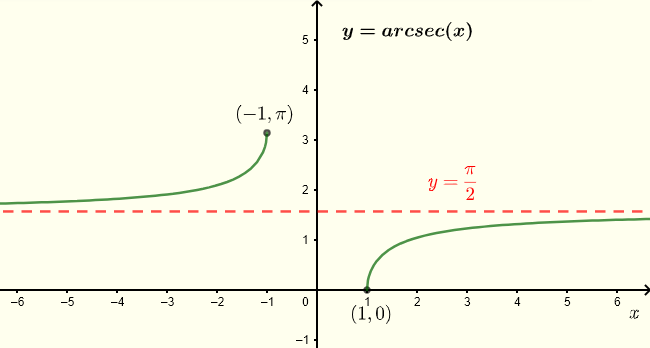# Arcsec(x) Calculator

An online calculator to calculate inverse secant function arcsec(x) in radians and degrees. The graph of y = arcsec(x) is shown below and it has the horizontal asymptote $y = \dfrac{\pi}{2}$ defined as
$\lim_{x\to-\infty} arcsec(x) = \dfrac{\pi}{2}$      and      $\lim_{x\to 0} arcsec(x) = \dfrac{\pi}{2}$
The domain of y = arcsec(x) is given by:      $-\infty \lt x \le -1 \quad \cup \quad 1 \le x \lt \infty$
The range of y = arcsec(x) is given by:       $0 \le y \lt \dfrac{\pi}{2} \quad \cup \quad \dfrac{\pi}{2} \lt y \le \pi$## How to use the arcsec (x) calculator

1 - Enter x as a real number and the number of decimal places desired then press "enter". Two answers; one in radians and the other in degrees are displayed. For any value of x outside the domain defined above "NaN"(not a number) is displayed.

 x = 1 Decimal Places = 3 arcsec(x)= radians arcsec(x) = degrees

You may use it to explore the domain and range of arccot(x).

More Math Calculators and Solvers.